# Missing geom_point and timeseries marker from axis

I am trying multiple step time series and point mark overlapping found a bug notice the figure save the png are good but missing few thing in html

Also when i use matplot using to_bokeh its miss the time series

GGPLOT

http://bokeh.pydata.org/en/0.11.1/docs/gallery/step.html
=== Modified little pit added geom_point

from ggplot import aes, geom_step, ggplot , ggsave , geom_point
import matplotlib

import matplotlib.pyplot as plt
import numpy as np
import pandas as pd

from bokeh import mpl
from bokeh.plotting import output_file, show

plt.switch_backend(‘agg’)
matplotlib.use(‘Agg’)
df = pd.DataFrame({
“x”: range(100),
“y”: np.random.choice([-1, 1], 100)
})
df.y = df.y.cumsum()

g = ggplot(aes(x=‘x’, y=‘y’), data=df) + geom_step() + geom_point(aes(x=‘x’, y=‘y’) )
g.draw()
ggsave(plot=g, filename=“t.png” )

plt.title(“Step ggplot-based plot in Bokeh.”)

output_file("/tmp/ggplot_step.html", title=“ggplot_step.py example”)

show(mpl.to_bokeh())

PNG from ggsave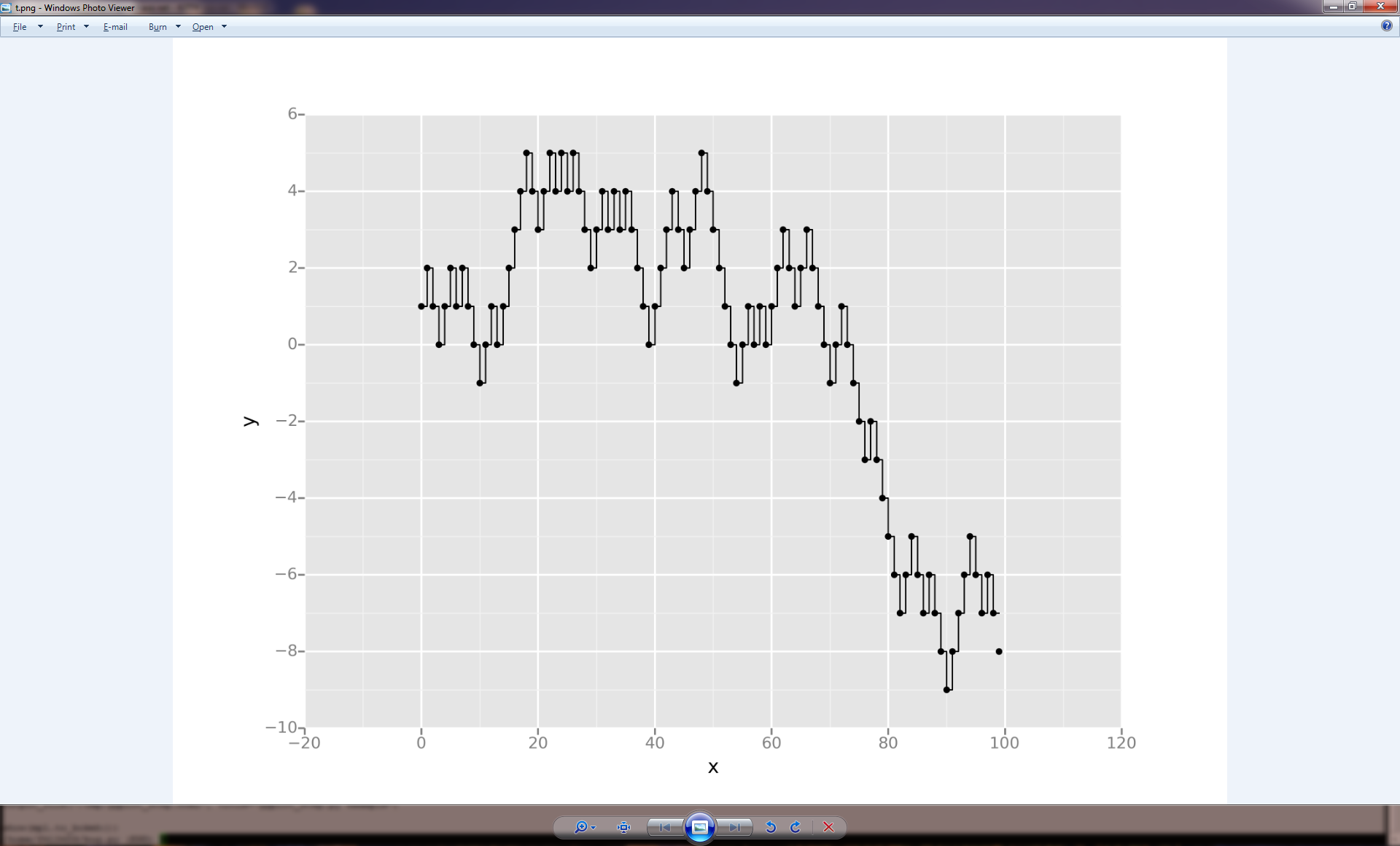html missing point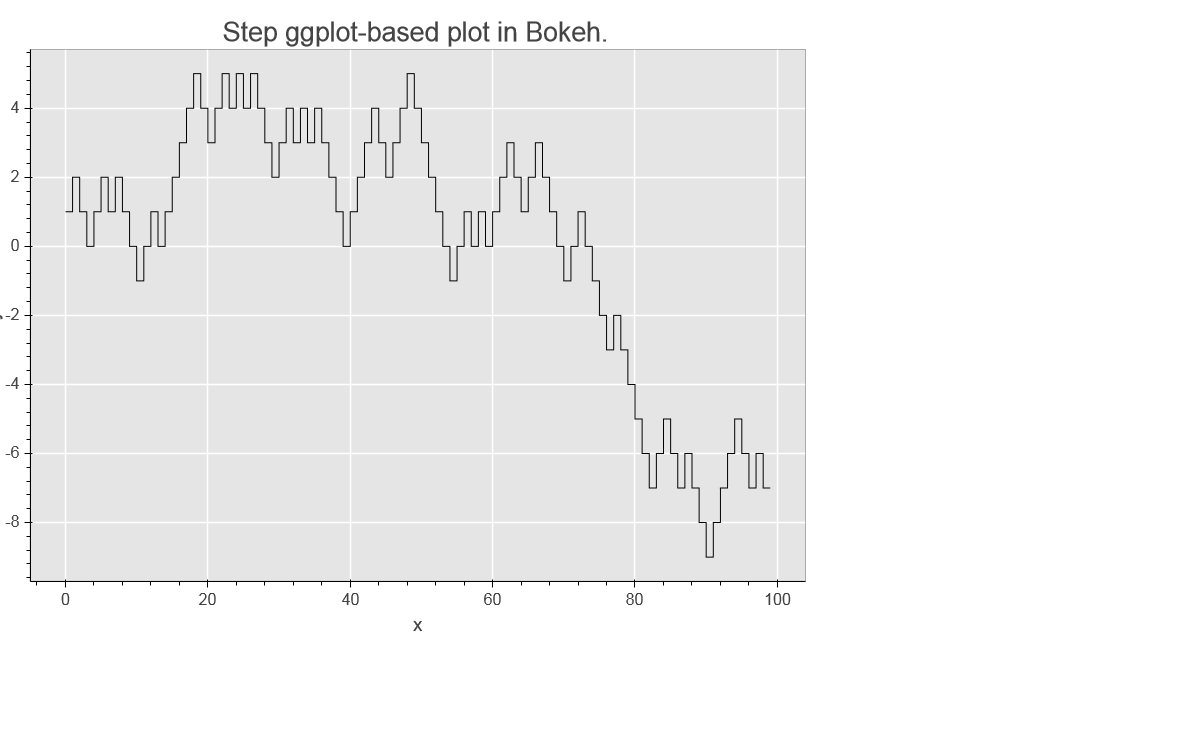from matplotlib to bokeh conversion

Missing timeseries format
step function is not exact its render as line some time
can have point on figure too

original figure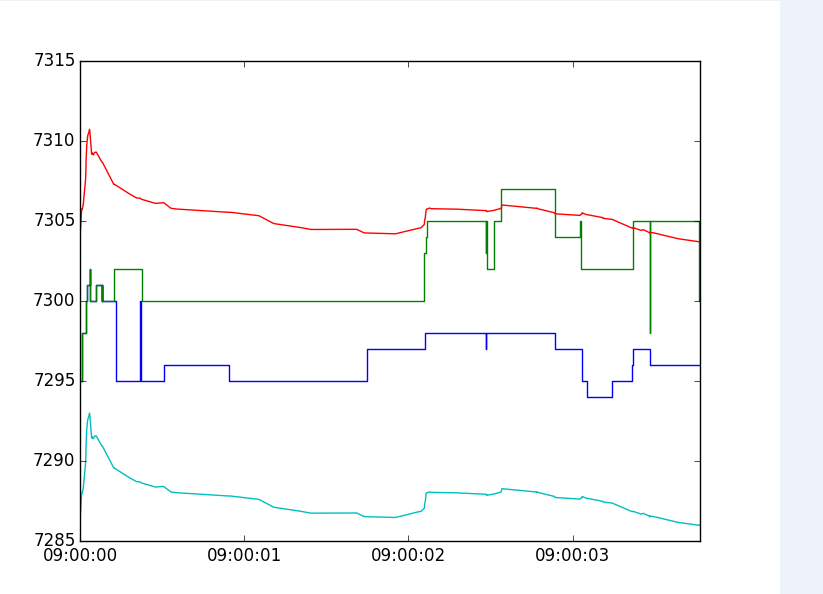html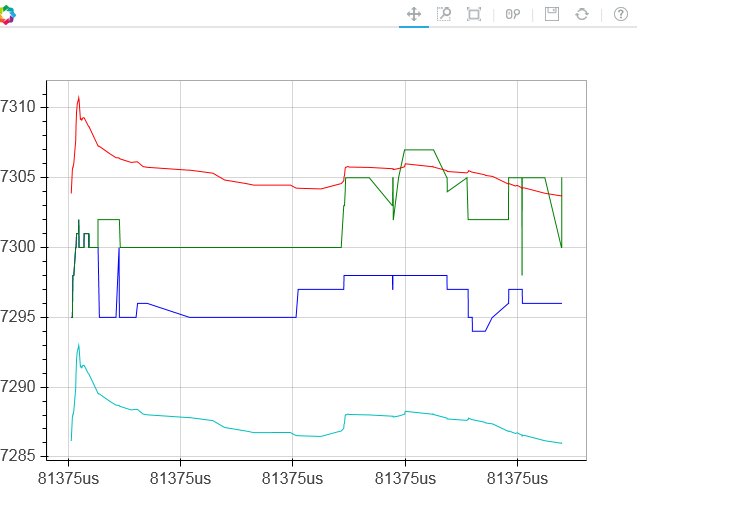What i want to do something below in HTML view may you can suggest some solution to have some step time series and few marker point on time series

-So for i tried timeseries with different builder doesn’t work
-ggplot step function good but missing the point time series is stilll wrong
-matplotlib also same issue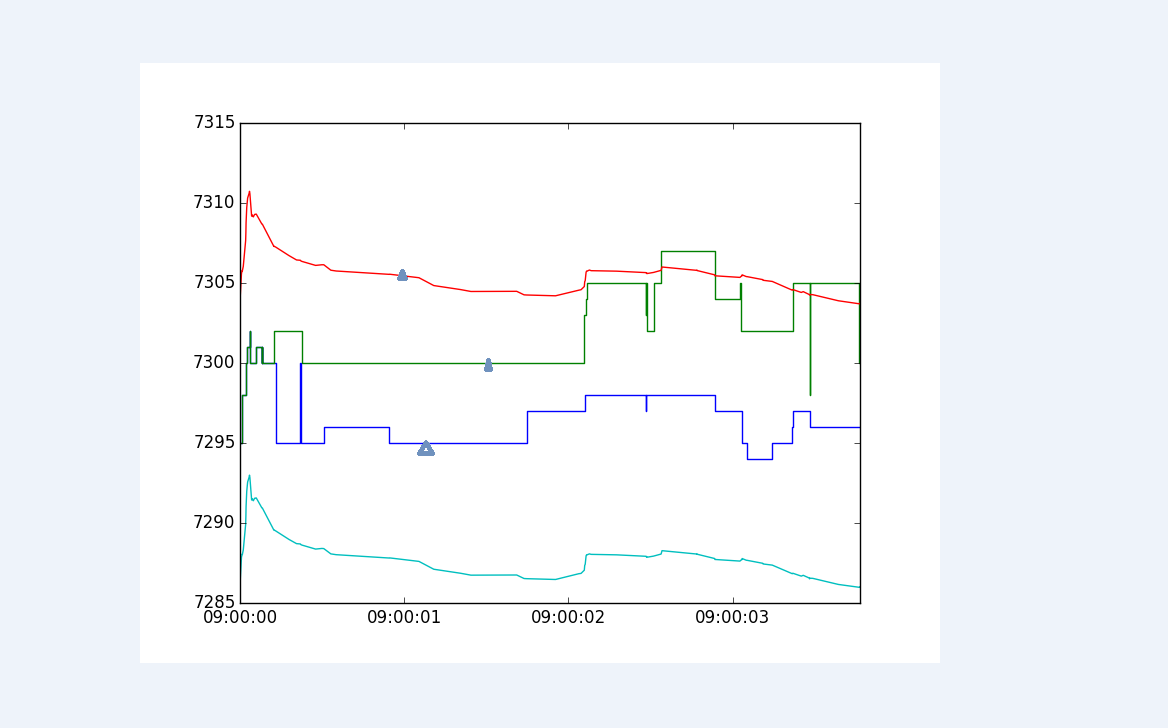Hi,

The library that Bokeh uses to help provide MPL compatibility is fairly useful, but it is definitely not complete. Better MPL compatibility is waiting on MEP 25 (https://github.com/matplotlib/matplotlib/wiki/MEP25) but I can't speculate when that work might be done. Please make a GH issue on the Bokeh issue tracker (https://github.com/bokeh/bokeh/issues) so that these problems can possibly by re-visited at at a later date when better MPL compatibility is available. In the mean time, I'd suggest using native Bokeh APIs directly when possible, for the best results.

Bryan

···

On May 3, 2016, at 2:17 AM, [email protected] wrote:

I am trying multiple step time series and point mark overlapping found a bug notice the figure save the png are good but missing few thing in html

Also when i use matplot using to_bokeh its miss the time series

GGPLOT

http://bokeh.pydata.org/en/0.11.1/docs/gallery/step.html
=== Modified little pit added geom_point

from ggplot import aes, geom_step, ggplot , ggsave , geom_point
import matplotlib

import matplotlib.pyplot as plt
import numpy as np
import pandas as pd

from bokeh import mpl
from bokeh.plotting import output_file, show

plt.switch_backend('agg')
matplotlib.use('Agg')
df = pd.DataFrame({
"x": range(100),
"y": np.random.choice([-1, 1], 100)
})
df.y = df.y.cumsum()

g = ggplot(aes(x='x', y='y'), data=df) + geom_step() + geom_point(aes(x='x', y='y') )
g.draw()
ggsave(plot=g, filename="t.png" )

plt.title("Step ggplot-based plot in Bokeh.")

output_file("/tmp/ggplot_step.html", title="ggplot_step.py example")

show(mpl.to_bokeh())

PNG from ggsave

<Auto Generated Inline Image 1.png>

html missing point

<Auto Generated Inline Image 2.png>

from matplotlib to bokeh conversion

Missing timeseries format
step function is not exact its render as line some time
can have point on figure too

original figure

<Auto Generated Inline Image 3.png>
html

<Auto Generated Inline Image 4.png>

What i want to do something below in HTML view may you can suggest some solution to have some step time series and few marker point on time series

-So for i tried timeseries with different builder doesn't work
-ggplot step function good but missing the point time series is stilll wrong
-matplotlib also same issue

<Auto Generated Inline Image 5.png>

--
You received this message because you are subscribed to the Google Groups "Bokeh Discussion - Public" group.
To unsubscribe from this group and stop receiving emails from it, send an email to [email protected].
To post to this group, send email to [email protected].